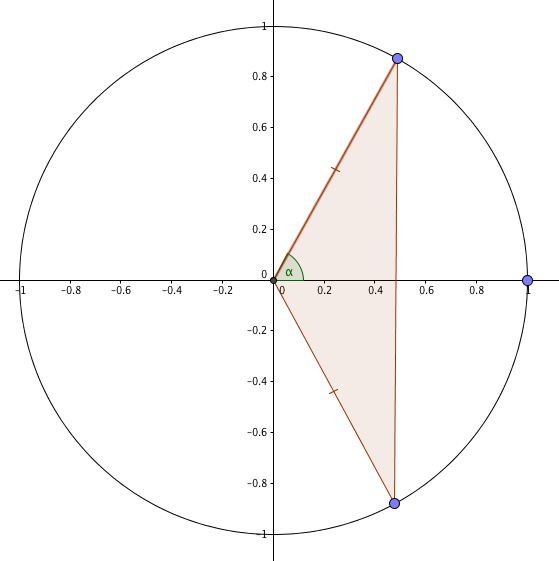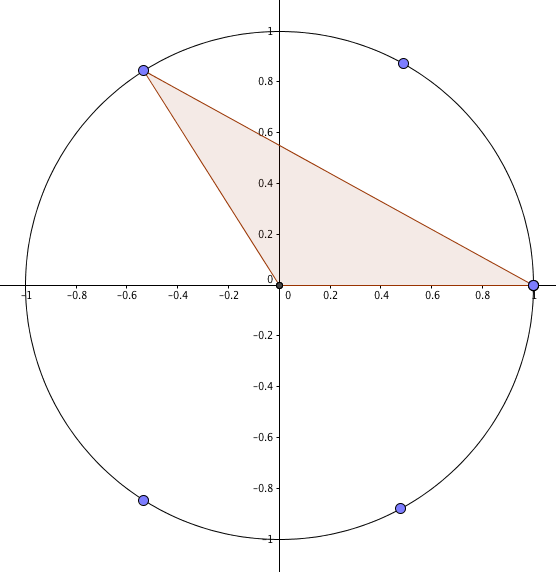Uncle Colin recently explained how he would prove the identity $\sin(2x) \equiv 2 \sin(x)\cos(x)$. Naturally, that isn’t the only proof.

@traumath pointed me at an especially elegant one involving the unit circle. Suppose we have an isosceles triangle set up like this:The vertical ‘base’ of the triangle is $2\sin(\alpha)$ units; the perpendicular ‘height’ is $\cos(\alpha)$, both easily established from right-angle trigonometry or (you know) the definitions of sine (roughly speaking, how far up the angle takes you on the unit circle) and cosine (how far across). Its area is $\sin(\alpha)\cos(\alpha)$.

Now suppose we rotate the triangle by an angle of $\alpha$ around the origin like so:The horizontal base of this triangle is one unit, and the perpendicular height is $\sin(2\alpha)$, making the area of the triangle $\frac 12 \sin(2\alpha)$.

We’ve only rotated it, so its area hasn’t changed – which means $\sin(\alpha)\cos(\alpha) \equiv \frac 12 \sin(2\alpha)$. Double everything and boom! There’s your identity.

* Edited 2016-08-25 to link to the previous post.